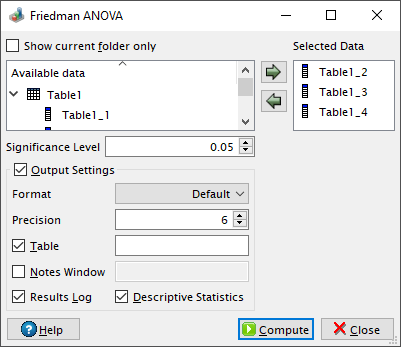Friedman ANOVA Dialog

This dialog is activated by selecting the Friedman ANOVA... command from the Statistics -> Nonparametric Tests -> menu. It can be used in order to test whether several samples originate from the same distribution. QtiPlot uses the test procedure explained in the following Wikipedia article.

Figure 5-92. The Friedman ANOVA dialog.The Friedman test is only applicable to complete data blocks, missing values are not accepted, therefore you need to check that the input data samples (table columns) have the same number of non-empty cells. The procedure involves ranking the scores in each input column with respect to the corresponding observations from the same table row. Average ranks are assigned to tied scores.

QtiPlot computes the test statistic (Q) and an approximated p-value. The approximation is based on the fact that when the size of the input samples (n) or the number of samples (k) is large (i.e. n > 15 or k > 4), the probability distribution of Q is that of a chi-squared distribution whith k-1 degrees of freedom (DoF). The p-value computed by QtiPlot is the probability of a chi-squared value of at least Q being observed: 1-chi2cdf(Q, k-1), where the chi2cdf function calculates the lower tail of the cumulative distribution function for the chi-squared distribution. The null hypothesis is rejected if the approximated probability is lower than the value of the significance level.

The data sets selected in the Available data window can be added to the analysis either by pressing the left to right arrow button or by dragging and dropping them onto the right side window.